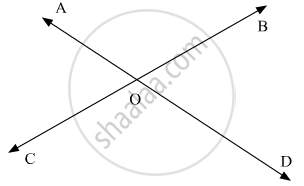# Consider the Following Statements: When Two Straight Lines Intersect: (I) Adjacent Angles Are Complementary (Ii) Adjacent Angles Are Supplementary - Mathematics

MCQ

Consider the following statements:
When two straight lines intersect:
(iii) opposite angles are equal
(iv) opposite angles are supplementary

Of these statements

#### Options

•  (i) and (ii) are correct

• (ii) and (iii) are correct

•  (i) and (iv) are correct

• (ii) and (iv)  are correct

#### Solution

Let us draw the following diagram showing two straight lines AD and BC intersecting each other at a point O.Now, let us consider each statement one by one:

(i) When two lines intersect adjacent angles are complementary.

This statement is incorrect

Explanation:

As the adjacent angles form a linear pair and they are supplementary.

(ii) When two lines intersect adjacent angles are supplementary.

This statement is correct.

Explanation:

As the adjacent angles form a linear pair and they are supplementary.

(iii) When two lines intersect opposite angles are equal.

This statement is correct.

Explanation:

As the vertically opposite angles are equal.

(iv) When two lines intersect opposite angles are supplementary.

This statement is incorrect.

Explanation:

As the vertically opposite angles are equal

Thus, out of all, (ii) and (iii) are correct.

Concept: Concept of Parallel Lines
Is there an error in this question or solution?

#### APPEARS IN

RD Sharma Mathematics for Class 9
Chapter 10 Lines and Angles
Q 4 | Page 51